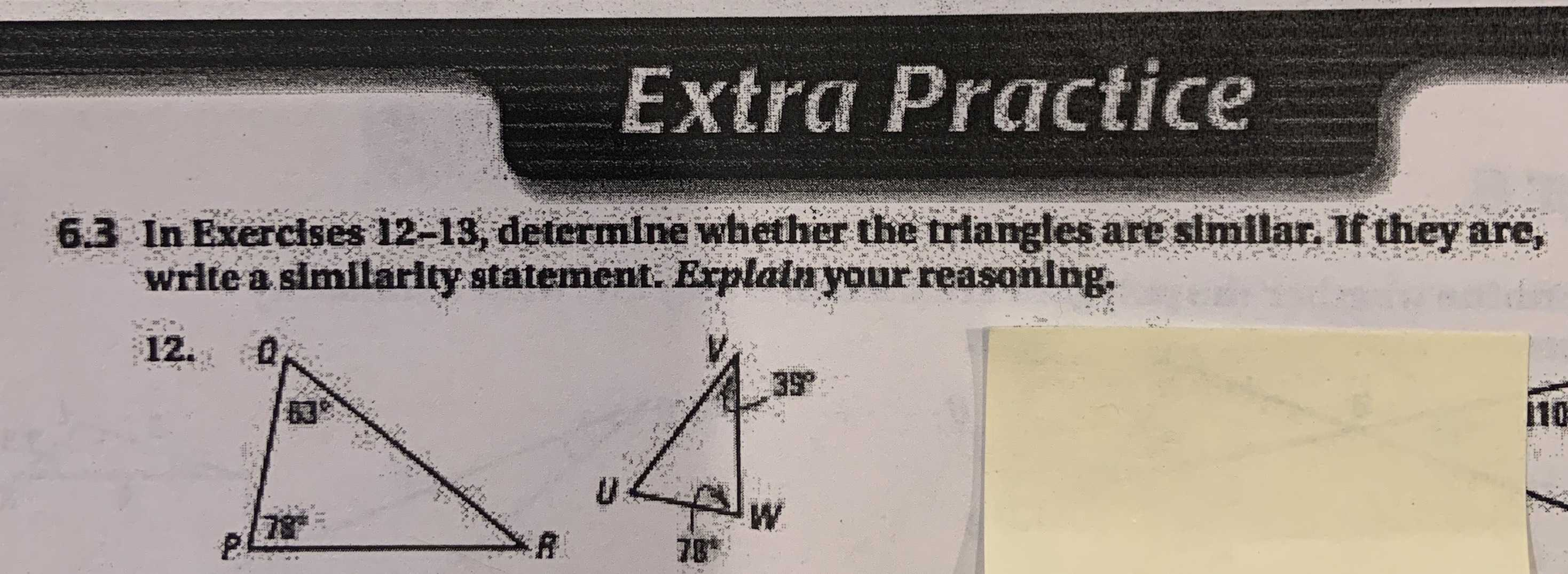### Still have math questions?

Trigonometry
Question$$63$$ In Exercfses $$12 - 13$$ , defernline whetlier the tringles art similar If they are, wrtea similarly statement Eoplamyour reasoning.

$$\frac{QP}{UW} = \frac{PR}{WV} = \frac{QR}{UV}$$